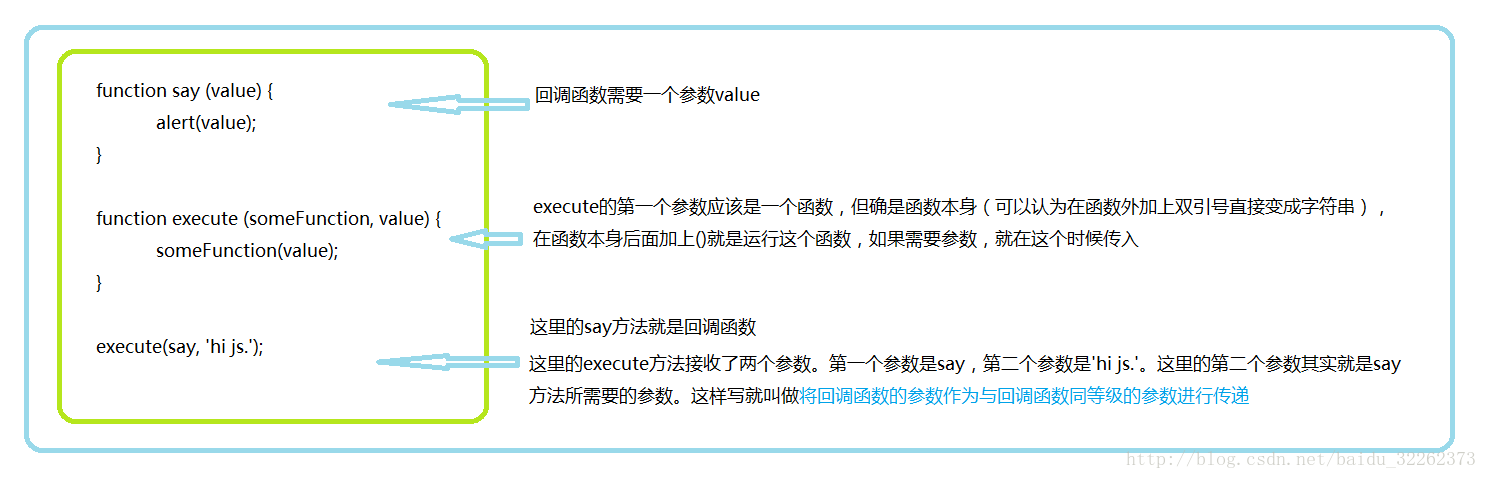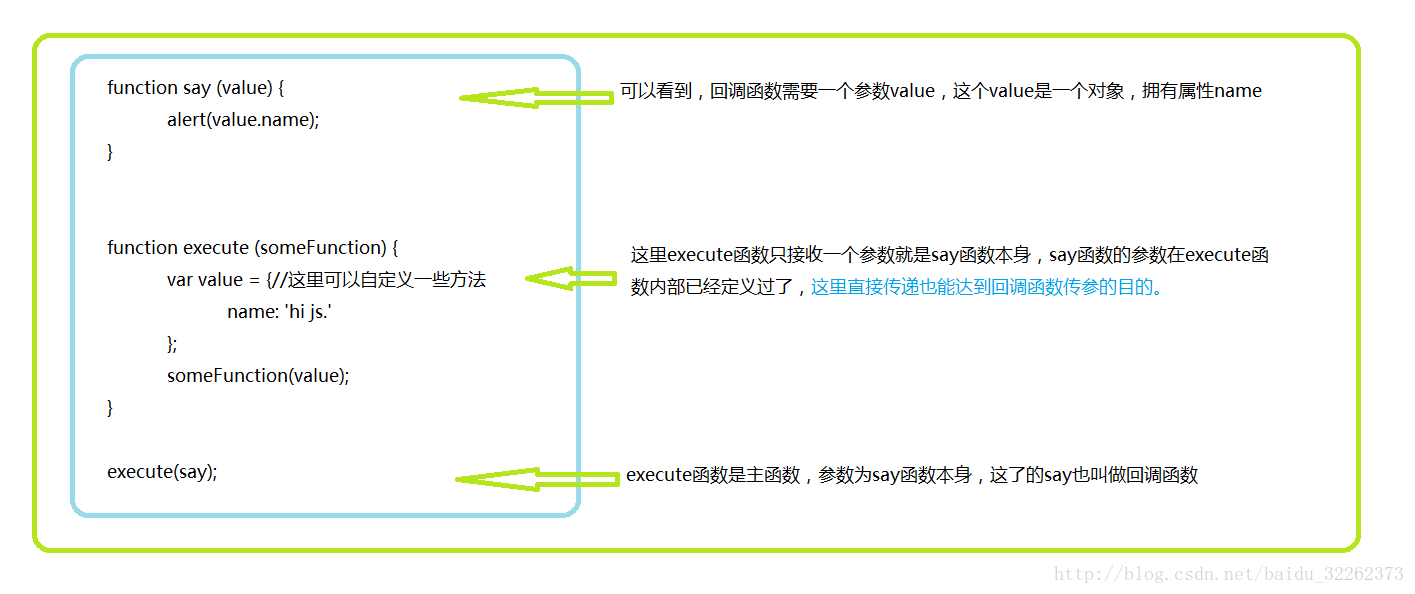### 一、前奏

``````function say (value) {
}
``````

``````只写变量名  say   返回的将会是 say方法本身，以字符串的形式表现出来。

``````

### 二、js中函数可以作为参数传递

``````function say (value) {
}
function execute (someFunction, value) {
someFunction(value);
}
execute(say, 'hi js.');
``````

``````function execute (someFunction, value) {
someFunction(value);
}
``````

``````function say (value) {
}
// 注意看下面,直接写say方法的方法名与下面的匿名函数可以认为是一个东西
// 这样再看上面两段代码是不是对函数可以作为参数传递就更加清晰了
say;

function (value) {
}
``````
``````这里的say或者匿名函数就被称为回调函数。
``````————————————————

### 四、其他介绍

``````	<script type="text/javascript">
let sum = a + b;
fun(sum);
}

function log(sum) {
document.write("我是方法1,拿到的数字是:" + sum+"</br>")
}
//方法1

//方法2: 内嵌函数方法:
document.write("我是方法2,拿到的数字是:" + sum)
})
</script>
``````## 详解

javascript 函数也是一种变量可以成为其他函数的参数, 我们喜欢在js中称之为回调函数, 另外js中的匿名函数可以作为函数参数使得回调函数的写法如鱼得水.

ps. 回调函数与 c 语言中的函数指针类似—指针指针指针!!!重要的事情说三遍

## 回调函数

``````  function addSqua(num1, num2, callback){
var sum = num1 + num2;
return callback(sum);
}

function squa(num){
return num*num;
}

let num = addSqua(1, 2, squa);
console.log(num);
//=>9
``````

## 匿名回调函数

``````function addSqua(num1, num2, callback){
var sum = num1 + num2;
return callback(sum);
}
let num = addSqua(1, 2, function squa(num){
return num*num;
});
console.log(num);
//=9
``````

*上面的function squa(num){}函数，可以写成function (num){}匿名函数

## 模仿数组中的every方法

``````  Array.prototype.myEvery = function (callback) {
if (typeof callback === 'function') {
for (let i = 0; i < this.length; i++) {
if (callback(this[i])) {
return false;
}
}
return true;
}
return true;
}

let op = [3, 6, 'every', 9,'each'];
op.myEvery(function (value) {
console.log(value);
})
// 3
// 6
// 'every'
// 9
// 'each'
``````

## 回调函数特点

• 不会立刻执行, 正如定义中给出的一样回调函数作为代码片段可以根据需要在其特定条件下执行, 回调函数作为参数传递给一个函数的时候，传递的只是函数的定义并不会立即执行。
• this，ES6推出了箭头函数, 箭头函数的推出需要在写回调函数的时候格外注意, this 是指向所在函数的上下文对象, 如果在在ES6采用箭头函数则需要注意 this使用, 箭头函数中this并没有上下文关系直说, 有兴趣的可以查看ES6 阮一峰, 再次奉上传送门
回调函数的优点和使用场景是个闭包

## 回调函数之美

-DRY，避免重复代码。
-可以将通用的逻辑抽象。
-业务逻辑分离 (it’s so beautiful - -)
-提高代码可维护性和可读性。
-加强代码可读性。
-分离专职的函数。
js回调函数的伟大之处是其他传统语言所没有的, 它可以实现业务逻辑分离, 相当于暴露给外界一个接口, 这一点像极了前后端分离架构中的 API 接口设计理念

*知识点收集与网络